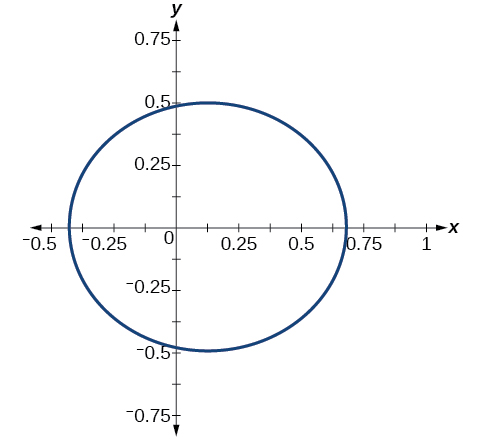# 8.5 Conic sections in polar coordinates  (Page 3/8)

 Page 3 / 8

## Graphing a hyperbola in polar form

Graph

First, we rewrite the conic in standard form by multiplying the numerator and denominator by the reciprocal of 2, which is $\text{\hspace{0.17em}}\frac{1}{2}.$

Because $\text{\hspace{0.17em}}e=\frac{3}{2},e>1,$ so we will graph a hyperbola    with a focus at the origin. The function has a term and there is a subtraction sign in the denominator, so the directrix is $\text{\hspace{0.17em}}y=-p.$

The directrix is $\text{\hspace{0.17em}}y=-\frac{8}{3}.$

Plotting a few key points as in [link] will enable us to see the vertices. See [link] .

A B C D
$\theta$ $0$ $\frac{\pi }{2}$ $\pi$ $\frac{3\pi }{2}$
$r=\frac{8}{2-3\mathrm{sin}\text{\hspace{0.17em}}\theta }$ $4$ $-8$ $4$ $\frac{8}{5}=1.6$

## Graphing an ellipse in polar form

Graph

First, we rewrite the conic in standard form by multiplying the numerator and denominator by the reciprocal of 5, which is $\text{\hspace{0.17em}}\frac{1}{5}.$

Because $\text{\hspace{0.17em}}e=\frac{4}{5},e<1,$ so we will graph an ellipse    with a focus at the origin. The function has a $\text{\hspace{0.17em}}\text{cos}\text{\hspace{0.17em}}\theta ,$ and there is a subtraction sign in the denominator, so the directrix    is $\text{\hspace{0.17em}}x=-p.$

The directrix is $\text{\hspace{0.17em}}x=-\frac{5}{2}.$

Plotting a few key points as in [link] will enable us to see the vertices. See [link] .

A B C D
$\theta$ $0$ $\frac{\pi }{2}$ $\pi$ $\frac{3\pi }{2}$
$10$ $2$ $\frac{10}{9}\approx 1.1$ $2$

Graph## Deﬁning conics in terms of a focus and a directrix

So far we have been using polar equations of conics to describe and graph the curve. Now we will work in reverse; we will use information about the origin, eccentricity, and directrix to determine the polar equation.

Given the focus, eccentricity, and directrix of a conic, determine the polar equation.

1. Determine whether the directrix is horizontal or vertical. If the directrix is given in terms of $\text{\hspace{0.17em}}y,$ we use the general polar form in terms of sine. If the directrix is given in terms of $\text{\hspace{0.17em}}x,$ we use the general polar form in terms of cosine.
2. Determine the sign in the denominator. If $\text{\hspace{0.17em}}p<0,$ use subtraction. If $\text{\hspace{0.17em}}p>0,$ use addition.
3. Write the coefficient of the trigonometric function as the given eccentricity.
4. Write the absolute value of $\text{\hspace{0.17em}}p\text{\hspace{0.17em}}$ in the numerator, and simplify the equation.

## Finding the polar form of a vertical conic given a focus at the origin and the eccentricity and directrix

Find the polar form of the conic given a focus at the origin, $\text{\hspace{0.17em}}e=3\text{\hspace{0.17em}}$ and directrix     $\text{\hspace{0.17em}}y=-2.$

The directrix is $\text{\hspace{0.17em}}y=-p,$ so we know the trigonometric function in the denominator is sine.

Because $\text{\hspace{0.17em}}y=-2,–2<0,$ so we know there is a subtraction sign in the denominator. We use the standard form of

and $\text{\hspace{0.17em}}e=3\text{\hspace{0.17em}}$ and $\text{\hspace{0.17em}}|-2|=2=p.$

Therefore,

## Finding the polar form of a horizontal conic given a focus at the origin and the eccentricity and directrix

Find the polar form of a conic given a focus at the origin, $\text{\hspace{0.17em}}e=\frac{3}{5},$ and directrix     $\text{\hspace{0.17em}}x=4.$

Because the directrix is $\text{\hspace{0.17em}}x=p,$ we know the function in the denominator is cosine. Because $\text{\hspace{0.17em}}x=4,4>0,$ so we know there is an addition sign in the denominator. We use the standard form of

and $\text{\hspace{0.17em}}e=\frac{3}{5}\text{\hspace{0.17em}}$ and $\text{\hspace{0.17em}}|4|=4=p.$

Therefore,

$\begin{array}{l}\begin{array}{l}\hfill \\ \hfill \\ r=\frac{\left(\frac{3}{5}\right)\left(4\right)}{1+\frac{3}{5}\text{\hspace{0.17em}}\mathrm{cos}\text{\hspace{0.17em}}\theta }\hfill \end{array}\hfill \\ r=\frac{\frac{12}{5}}{1+\frac{3}{5}\text{\hspace{0.17em}}\mathrm{cos}\text{\hspace{0.17em}}\theta }\hfill \\ r=\frac{\frac{12}{5}}{1\left(\frac{5}{5}\right)+\frac{3}{5}\text{\hspace{0.17em}}\mathrm{cos}\text{\hspace{0.17em}}\theta }\hfill \\ r=\frac{\frac{12}{5}}{\frac{5}{5}+\frac{3}{5}\text{\hspace{0.17em}}\mathrm{cos}\text{\hspace{0.17em}}\theta }\hfill \\ r=\frac{12}{5}\cdot \frac{5}{5+3\text{\hspace{0.17em}}\mathrm{cos}\text{\hspace{0.17em}}\theta }\hfill \\ r=\frac{12}{5+3\text{\hspace{0.17em}}\mathrm{cos}\text{\hspace{0.17em}}\theta }\hfill \end{array}$

Find the polar form of the conic given a focus at the origin, $\text{\hspace{0.17em}}e=1,$ and directrix $\text{\hspace{0.17em}}x=-1.$

$r=\frac{1}{1-\mathrm{cos}\theta }$

how do I set up the problem?
what is a solution set?
Harshika
hello, I am happy to help!
Abdullahi
find the value of 2x=32
divide by 2 on each side of the equal sign to solve for x
corri
X=16
Michael
Want to review on complex number 1.What are complex number 2.How to solve complex number problems.
Beyan
use the y -intercept and slope to sketch the graph of the equation y=6x
how do we prove the quadratic formular
hello, if you have a question about Algebra 2. I may be able to help. I am an Algebra 2 Teacher
thank you help me with how to prove the quadratic equation
Seidu
may God blessed u for that. Please I want u to help me in sets.
Opoku
what is math number
4
Trista
x-2y+3z=-3 2x-y+z=7 -x+3y-z=6
Need help solving this problem (2/7)^-2
x+2y-z=7
Sidiki
what is the coefficient of -4×
-1
Shedrak
the operation * is x * y =x + y/ 1+(x × y) show if the operation is commutative if x × y is not equal to -1
An investment account was opened with an initial deposit of \$9,600 and earns 7.4% interest, compounded continuously. How much will the account be worth after 15 years?
lim x to infinity e^1-e^-1/log(1+x)
given eccentricity and a point find the equiationByByBy Brooke DelaneyBy David GeltnerBy Vongkol HENGBy Mariah HauptmanBy Katy KeilersBy OpenStaxBy Edward BitonBy OpenStaxByBy Dan Ariely